# Using the flatness package, illustrated with weather ensemble forecasts

#### 29 June 2021

In this vignette we first motivate the existence of the flatness package and its most important implementation, the Jolliffe-Primo flatness tests. Then we illustrate its functionalities with data sets from the weather forecasting field.

# Motivation of the flatness package

## Limitations of the $$\chi^2$$ test

The flatness package is dedicated to the task of assessing and testing whether an histogram of counts in ordered and consecutive categories is flat, i.e. all the categories have the same count up to sampling noise.

The flatness of a histogram is traditionally tested with a $$\chi^2$$ statistical test, the null hypothesis being that the counts are evenly distributed between the categories up to sampling noise. Although this test is powerful it is not sensitive to some specific deviations from flatness. Let us illustrate this with a synthetic example, following Elmore (2005).

set.seed(42)
N <- 20
K <- 21
ranks <- sample(1:K, size = K * N, replace = TRUE)
shst <- hst <- hist(ranks, breaks = 0:K + 0.5)
abline(h = N, lty = 2)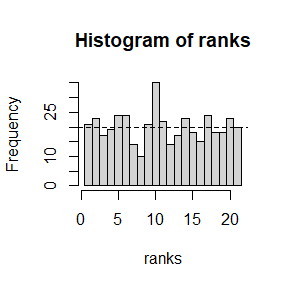shst$counts <- sort(shst$counts)
plot(shst, col ="lightgrey")
abline(h = N, lty = 2)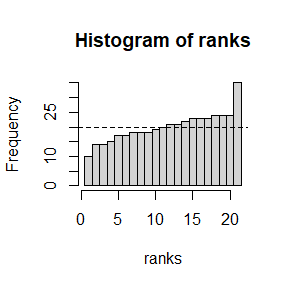cat("Random counts' chisquare statistics =", chisq.test(hst$counts)$statistic, "\n")
#> Random counts' chisquare statistics = 26.7
cat("Sorted counts' chisquare statistics =", chisq.test(shst$counts)$statistic, "\n")
#> Sorted counts' chisquare statistics = 26.7

We first randomly draw $$420$$ integers from a uniform distribution between 1 and $$K=21$$ then calculate the corresponding histogram hst. This one seems rather flat (i.e. counts are close to the expected theoretical value $$N = 20$$, drawn as the horizontal dashed line) judging from the first plot. Then we tweak the histogram by reordering the counts in ascending order to produce a blatantly unflat histogram shst. Despite the blatant sloping shape of the sorted histogram, as confirmed by the second plot, its $$\chi^2$$ test statistics is the same as the one for the first (truly) random histogram. Jolliffe and Primo (2008) proposed to decompose the $$\chi^2$$ test statistic in such a way that one can test the existence of such specific deviations to flatness in histograms.

## The Jolliffe-Primo flatness test

The principle of the Jolliffe-Primo test is the following (see Supplementary Material to Zamo et al., 2021). Let’s suppose a histogram with K possible ordered categories, each with count $$n_i$$ (with $$i=1,\ldots,K$$), and $$e$$ the theoretical count in each category of a perfectly flat histogram (i.e. $$e = \frac{\sum_{i=1}^Kn_i}{K}$$). Then the normalized deviation to flatness (i.e. Pearson residuals) of the histogram can be rewritten as a vector $\delta=\left(\frac{n_1 -e}{\sqrt{e}}, \ldots, \frac{n_K - e}{\sqrt{e}}\right).$ The squared norm of this vector is just the $$\chi^2$$ test statistics, which explains why it does not change if we permutate the counts (i.e. the components of vector $$\delta$$). This vector can then be projected on a set of up to $$K - 1$$ vectors $$u_j$$ (with $$j =1, \ldots, k \le K -1$$). It can be shown that, if these vectors are deviates (i.e. the sum of each vector’s components is zero) and the set is orthonormal, then the squared modules of the projections $$\delta \cdot u_j$$ each approximately and independently follows a $$\chi^2$$ distribution with 1 degree of liberty, under the null hypothesis of a flat rank histogram. By choosing appropriate deviate vectors $$u_j$$ we can test whether the shape corresponding to each deviate vector is present in a given histogram.

Let’s now describe the ensembles and ppensembles data sets (shipped with the flatness package) that will be used to illustrate how to use the flatness package and the Jolliffe-Primo flatness test.

# The ensembles and ppensembles datasets

## Ensembles and rank histograms

In Meteorology an ensemble forecast is a set of forecasts of the future state of the atmosphere. Each simulated evolution starts from a slightly different initial condition and use different evolution algorithm in order to represent the uncertainty of the future outcome. Each evolution is called a member. When forecasting a parameter if the ensemble forecast is a sample from the right multivariate probabilistic distribution of the outcome then the outcome and the members should be indistinguishable, so that any scalar outcome has the same probability to fall between any two consecutive forecast values. If one computes the rank of the observation when pooled with members and compute the count in each rank over several forecasts, then one should get a flat histogram up to sampling noise. This kind of histogram is called a rank histogram and its flatness can be assessed with scores and statistical tests.

## Contents of the ensembles datasets

Several meteorological institutions over the world issue their own ensemble forecasts, some of which are made freely available in the TIGGE ensemble. The ensembles dataset contains several ensemble forecasts for the 2-m temperature in Blagnac, France and the associated measured temperature.

First load the package and the ensembles dataset used for illustrative purpose.

library(flatness)
ensembles <- ensembles
is(ensembles)
#>  "list"   "vector"
names(ensembles)
#>  "CWAO" "DEMS" "ECMF" "EGRR" "RKSL"

We can see that the ensembles dataset is a named list with five entries. Each entry contains the ensemble forecast of one weather forecasting organization.

library(data.table)
ensembles$CWAO #> date_run latitude longitude 1 2 3 4 5 #> 1: 2019-03-01 43.5 1.5 280.3152 279.5232 276.6893 273.6942 276.5990 #> 2: 2019-03-02 43.5 1.5 274.8378 274.4615 272.9161 274.2175 273.5043 #> 3: 2019-03-03 43.5 1.5 280.1985 277.3259 278.9862 279.2702 279.2407 #> 4: 2019-03-04 43.5 1.5 275.4946 277.2073 275.6813 277.1495 277.5157 #> 5: 2019-03-05 43.5 1.5 278.4286 277.3543 277.7069 279.6607 280.0637 #> --- #> 727: 2021-03-27 43.5 1.5 271.2999 275.8482 272.9926 275.8783 273.1891 #> 728: 2021-03-28 43.5 1.5 276.4429 276.1473 277.9194 275.5068 277.0300 #> 729: 2021-03-29 43.5 1.5 279.0955 278.9508 280.1055 279.4067 279.1118 #> 730: 2021-03-30 43.5 1.5 278.0254 279.8665 280.5095 279.3302 279.6777 #> 731: 2021-03-31 43.5 1.5 280.3761 279.1144 279.3001 280.5096 280.3647 #> 6 7 8 9 10 11 12 13 #> 1: 279.3050 276.1084 279.8428 278.3054 281.2444 280.4360 277.3517 277.5993 #> 2: 274.4678 274.5705 274.7559 271.8764 273.9752 274.1097 273.8252 274.4106 #> 3: 278.0438 279.4622 283.5233 279.3260 282.0111 280.5855 283.0036 278.2077 #> 4: 277.1346 272.6506 276.4795 275.6070 277.4290 277.7994 274.1130 275.0794 #> 5: 277.6463 279.2020 279.4067 277.3104 278.9702 277.8915 278.2042 275.6957 #> --- #> 727: 275.3884 273.5678 272.3508 273.5719 273.1230 274.1035 275.2148 273.4731 #> 728: 278.3895 276.4772 277.2625 276.9016 276.2067 277.9131 276.5159 278.6302 #> 729: 278.4906 279.4412 278.8210 278.7421 278.8204 280.5871 279.1756 278.3197 #> 730: 280.0230 279.3890 280.0062 278.7466 280.5102 279.4570 279.5275 279.0752 #> 731: 280.0109 280.9742 280.3582 279.1833 280.6151 279.7941 281.9291 279.8622 #> 14 15 16 17 18 19 20 T #> 1: 280.1643 274.8325 279.2591 279.1006 279.0816 282.2659 278.7608 280.95 #> 2: 274.8890 274.8842 272.5728 275.1929 273.7774 274.2302 274.6507 276.15 #> 3: 281.3155 279.8067 278.5925 279.2088 278.9522 284.1115 281.8102 284.55 #> 4: 278.2044 277.3690 277.7078 275.6547 277.7249 276.3633 277.5169 279.95 #> 5: 276.7913 277.5009 279.4303 278.3975 279.3692 278.8182 279.2550 284.65 #> --- #> 727: 273.8149 273.0809 272.4609 273.9666 271.8731 272.7964 273.0863 276.45 #> 728: 278.3615 278.3091 277.5695 277.5956 277.7396 276.8420 278.2090 282.35 #> 729: 279.0861 279.4996 280.1250 278.9508 279.7057 278.2358 279.3305 283.05 #> 730: 278.8851 280.7101 280.1661 279.9876 279.1747 279.9753 280.6875 283.15 #> 731: 279.3304 279.2093 279.5693 278.7148 279.0761 279.7060 279.3638 283.65 range(ensembles$CWAO$date_run) #>  "2019-03-01" "2021-03-31" sapply(ensembles, function(x) NCOL(x) - 4) #> CWAO DEMS ECMF EGRR RKSL #> 20 11 50 17 24 Each ensemble forecast is stored as a data.table and contains several entries date_run the date of the initial condition from which the ensemble starts. The run time is 00TC and the lead time 30h, so that each forecast is valid for the following day at 6UTC. Each ensemble covers two years with one forecast per day, from March 2019 to March 2021 (731 dates, October 2019 being missing). latitude, longitude latitude and longitude of the nearest grid-point to the weather station (in °) 1 … M the forecast temperature for each member (in Kelvins). Note the number M of members varies from one ensemble to the other (from 11 for the DEMS ensemble up to 50 for the ECMF ensemble). Actually each ensemble has one more member, called the control member, not included in this data set T the 2-m height temperaure (in Kelvins) measured at the Toulouse-Blagnac weather station in France. ## Contents of the ppensembles datasets As we will see below ensembles have flaws that make their forecast probabilities unactionable for decision making. But since these forecast error are not completely random one can use machine learning methods to extract information from past forecast/observation archive and correct those errors. For ensemble forecast a much-used method is non homogeneous regression (NHR) introduced by Gneiting et al. (2005). In a nutshell NHR posits that the forecast parameter obeys some chosen probability distribution whose parameters depend on the ensemble statistics. The relationship between the probability distribution’s parameters and the ensemble statistics is fitted with likelihood maximization for instance. The ensembles dataset has been post-processed with NHR by supposing the forecast temperature follows a Gaussian distribution whose mean and standard deviation linearly depends on the ensemble mean and standard deviation respectively. The regression coefficients have been adjusted by minimizing the CRPS over a 60-day sliding window. The ppensembles dataset has the same structure as the ensembles dataset with the “members” being a 30-sample from the forecast distribution. ppensembles <- ppensembles print(names(ppensembles)) #>  "CWAO" "DEMS" "ECMF" "EGRR" "RKSL" print(str(ppensembles$ECMF))
#> Classes 'data.table' and 'data.frame':   731 obs. of  34 variables:
#>  $date_run : Date, format: "2019-03-01" "2019-03-02" ... #>$ latitude : num  43.5 43.5 43.5 43.5 43.5 43.5 43.5 43.5 43.5 43.5 ...
#>  $longitude: num 1.5 1.5 1.5 1.5 1.5 1.5 1.5 1.5 1.5 1.5 ... #>$ 1        : num  278 275 279 278 281 ...
#>  $2 : num 278 275 280 278 281 ... #>$ 3        : num  280 276 280 278 281 ...
#>  $4 : num 280 276 280 278 281 ... #>$ 5        : num  280 276 281 278 282 ...
#>  $6 : num 280 276 281 278 282 ... #>$ 7        : num  280 276 281 279 282 ...
#>  $8 : num 280 276 281 279 282 ... #>$ 9        : num  280 276 281 279 282 ...
#>  $10 : num 280 276 281 279 282 ... #>$ 11       : num  280 276 281 279 282 ...
#>  $12 : num 281 276 282 279 282 ... #>$ 13       : num  281 276 282 279 282 ...
#>  $14 : num 281 277 282 279 282 ... #>$ 15       : num  281 277 282 279 282 ...
#>  $16 : num 281 277 282 279 282 ... #>$ 17       : num  281 277 282 279 282 ...
#>  $18 : num 281 277 282 279 283 ... #>$ 19       : num  281 277 282 279 283 ...
#>  $20 : num 281 277 282 279 283 ... #>$ 21       : num  282 277 282 280 283 ...
#>  $22 : num 282 277 282 280 283 ... #>$ 23       : num  282 277 282 280 283 ...
#>  $24 : num 282 277 283 280 283 ... #>$ 25       : num  282 277 283 280 283 ...
#>  $26 : num 282 278 283 280 283 ... #>$ 27       : num  282 278 283 280 283 ...
#>  $28 : num 282 278 283 280 283 ... #>$ 29       : num  283 278 283 280 283 ...
#>  $30 : num 283 278 283 280 283 ... #>$ T        : num  281 276 285 280 285 ...
#>  - attr(*, ".internal.selfref")=<externalptr>
#> NULL
sapply(ppensembles, function(x) NCOL(x) - 4)
#> CWAO DEMS ECMF EGRR RKSL
#>   30   30   30   30   30

# Building and representing a rank histogram

The workhorse to build rank histograms is the S3 generic function rkhist. Let’s start with one rank histogram. We can build it by sending a forecast matrix and an observation vector into rkhist.

iobs <- which(names(ensembles$CWAO) == "T") ifcst <- 4:(iobs - 1) M <- length(ifcst) K <- M + 1 print(paste("Number of members:", M)) #>  "Number of members: 20" fcst <- data.matrix(ensembles$CWAO[, ifcst, with = FALSE])
#>   ..$: NULL #> NULL plot(deviates)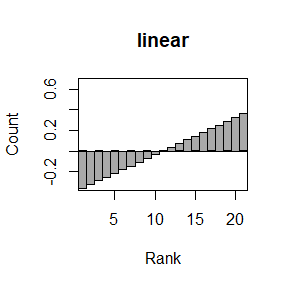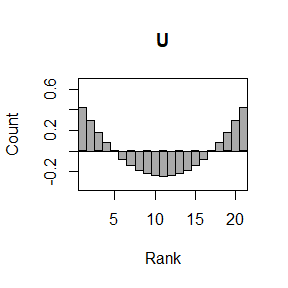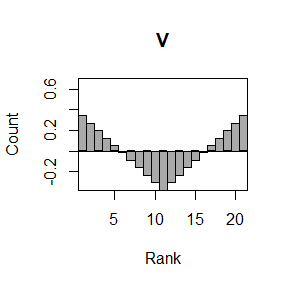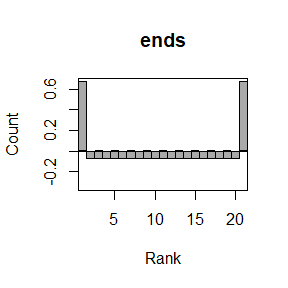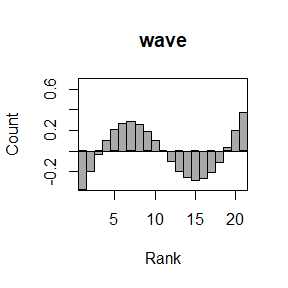Function get_deviates requires the number of possible categories (k) and the required shapes (shapes), and returns an rkhist object with one deviate by row for each requires shape. Internally this function calls a function named deviate_XXX for each required shape in shapes. This allows the user to easily implements its own deviate by following this naming convention to code its own function returning an rkhist object. ## Checking and imposing the requirements for the Jolliffe-Primo flatness test As stated above the Jolliffe-Primo flatness test requires a set of deviate vectors such that the set is orthonormal. This can be checked with function is_JP_ready. check <- is_JP_ready(x = deviates, tol = 0.001) #> Vector set is not OK (tolerance is: 0.001) #> Cross product should be unit diagonal: #> linear U V ends wave #> linear 1.000000e+00 -2.775558e-17 1.387779e-17 0.000000e+00 0.000000e+00 #> U -2.775558e-17 1.000000e+00 9.671773e-01 6.286946e-01 -2.775558e-16 #> V 1.387779e-17 9.671773e-01 1.000000e+00 5.085586e-01 -2.220446e-16 #> ends 0.000000e+00 6.286946e-01 5.085586e-01 1.000000e+00 -1.665335e-16 #> wave 0.000000e+00 -2.775558e-16 -2.220446e-16 -1.665335e-16 1.000000e+00 #> Row sums should be null: #> linear U V ends wave #> 0.000000e+00 2.602085e-16 -4.163336e-17 2.081668e-16 -2.719559e-15 print(str(check)) #> List of 5 #>$ isOK     : logi FALSE
#>  $tol : num 0.001 #>$ crossprod: num [1:5, 1:5] 1.00 -2.78e-17 1.39e-17 0.00 0.00 ...
#>   ..- attr(*, "dimnames")=List of 2
#>   .. ..$: chr [1:5] "linear" "U" "V" "ends" ... #> .. ..$ : chr [1:5] "linear" "U" "V" "ends" ...
#>  $sums : Named num [1:5] 0.00 2.60e-16 -4.16e-17 2.08e-16 -2.72e-15 #> ..- attr(*, "names")= chr [1:5] "linear" "U" "V" "ends" ... #>$ x        : 'rkhist' num [1:5, 1:21] -0.36 0.423 0.342 0.673 -0.373 ...
#>   ..- attr(*, "dimnames")=List of 2
#>   .. ..$: chr [1:5] "linear" "U" "V" "ends" ... #> .. ..$ : NULL
#> NULL

This function requires as argument a matrix x containing the checked vectors (one vector in each row). It checks whether the sum of components of each vector is 0 and the set of vectors is orthonormal. The optional argument tol gives a tolerance on the conditions. is_JP_ready returns a named list with the following entries

isOK
a logical. Are both requirements true?
tol
a numeric. The tolerance used to check the requirements.
crossprod
a matrix. This is the cross product between the row-vectors of the input matrix
sums
a named numeric vector containing the sum of components of each row-vector in the input matrix
x
the input vectors

Here we can see that the set of vectors deviates do not meet the requirements for the Jolliffe-Primo test due to the deviates U, V and ends being not orthogonal. One can use the function make_JP_ready to enforce the requirements on a set of vectors.

deviates_ok <- make_JP_ready(x = deviates)
#> Vector set is not OK (tolerance is: 1e-04)
#> Cross product should be unit diagonal:
#>               linear             U             V          ends          wave
#> linear  1.000000e+00 -2.775558e-17  1.387779e-17  0.000000e+00  0.000000e+00
#> U      -2.775558e-17  1.000000e+00  9.671773e-01  6.286946e-01 -2.775558e-16
#> V       1.387779e-17  9.671773e-01  1.000000e+00  5.085586e-01 -2.220446e-16
#> ends    0.000000e+00  6.286946e-01  5.085586e-01  1.000000e+00 -1.665335e-16
#> wave    0.000000e+00 -2.775558e-16 -2.220446e-16 -1.665335e-16  1.000000e+00
#> Row sums should be null:
#>        linear             U             V          ends          wave
#>  0.000000e+00  2.602085e-16 -4.163336e-17  2.081668e-16 -2.719559e-15
#> Vector set is OK (tolerance is: 1e-04)
plot(deviates_ok)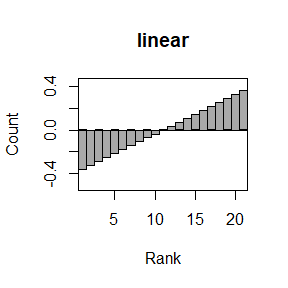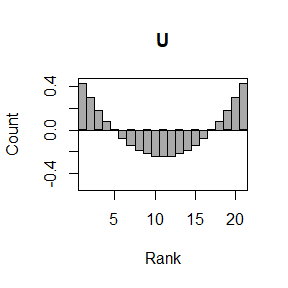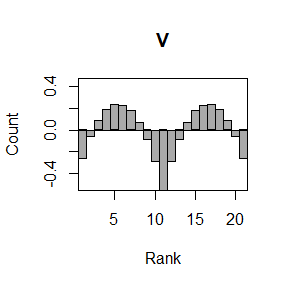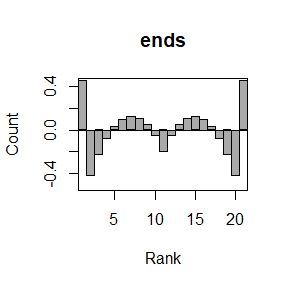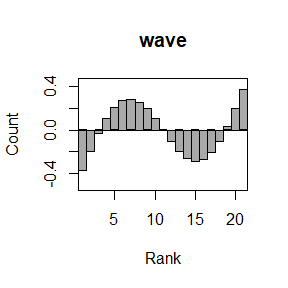Function make_JP_ready returns a vector set that can be used in the Jolliffe-Primo flatness test, by using the Grahm-Schmidt method on the input set (if necessary) and normalizing the sum of each vector’s components to zero (if necessary). But as can be noted from the plots above the shapes in the returned set may differ a lot from the ones in the inputted vector set (compare the histogram for V and ends with the previous plot). It is thus strongly advised to check visually that the deviates returned by this function really match the shapes the user wants to test the presence of in the histograms.

In the following we use the default set of deviate vectors c("linear", "U", "wave").

deviates <- get_deviates(k = K)
print(row.names(deviates))
#>  "linear" "U"      "wave"

## Applying the Jolliffe-Primo flatness test

Function JP_test implements the Jolliffe-Primo flatness test. It is an S3 generic function that requires two arguments rkhists (the histograms to be tested) and deviates (the matrix of deviates, with one deviate in each row).

JP <- JP_test(rkhists = rkh, deviates = deviates)
print(str(JP))
#> List of 3
#>  $deviates:List of 1 #> ..$ : 'rkhist' num [1:3, 1:21] -0.36 0.423 -0.373 -0.324 0.296 ...
#>   .. ..- attr(*, "dimnames")=List of 2
#>   .. .. ..$: chr [1:3] "linear" "U" "wave" #> .. .. ..$ : NULL
#>  $rkhists :List of 1 #> ..$ : 'rkhist' int [1, 1:21] 1 1 0 0 1 1 2 0 3 2 ...
#>   .. ..- attr(*, "dimnames")=List of 2
#>   .. .. ..$: chr "CWAO" #> .. .. ..$ : NULL
#>  $test : num [1:3, 1, 1:4] 241 58055 0 258 66442 ... #> ..- attr(*, "dimnames")=List of 3 #> .. ..$ result: chr [1:3] "projections" "statistics" "pvalues"
#>   .. ..$rkhist: chr "CWAO" #> .. ..$ test  : chr [1:4] "linear" "U" "wave" "resid."
#>  - attr(*, "class")= chr [1:2] "JPtest" "list"
#> NULL

The returned object by the implemented method is a named list with the following entries:

deviates
the set of $$S$$ deviates used as argument of the function
rkhists
the $$R$$ (rank) histograms used as argument of the function
test
this is a 3xRx(S+1) array containing the information necessary for the test.

The test entry has the following contents in each dimension:

projections
the projection of each rank histogram on each deviate
statistics
the corresponding $$\chi^2$$ test statistics
pvalues
the p-values computed from the test statistics

Let’s note that the last dimension of the test entry has length the number of deviates plus one. Indeed if the square of the projections of the histogram vector on each deviate follows a $$\chi^2$$ distribution with one degree of liberty, the module of the projection of the histogram vector on the complement of the vector space spanned by the set of deviates follow a $$\chi^2$$ distribution with $$K -S -1$$ degrees of liberty. Jp_test computes the test statistics and p-value for this complement vector. This allows to test whether deviations other than the one tested may be present in the rank histogram. But, as for the $$\chi^2$$ test on the initial histogram, this test is not powerful in detecting specific shapes. The name of this dimension in the test entry is resid. (for residuals).

Finally let’s note that this function does not actually perform the Jolliffe-Primo test but return the required information (p-values) to perform it. This implementation choice has been made because the flatness test is usually a multi-hypothesis statistical testing (several deviations are tested for several histograms), which requires corrections, such as the Bonferroni correction or the Benjamini-Hochberg procedure. The Benjamini-Hochberg procedure is implemented in function BH_procedure of the flatness package. See Benjamini and Hochberg (1995) for more details.

## Printing and plotting results

Function JP_test is an S3 generic function whose methods should return an object with S3 class JPtest. Corresponding methods for class JPtest of functions print and lattice::levelplot have been implemented.

print(JP)
#> Results of the Jolliffe-Primo test:
#> projections:
#>   linear        U     wave   resid.
#> 240.9467 257.7638 215.0518       NA
#> statistics:
#>    linear         U      wave    resid.
#>  58055.32  66442.15  46247.26 162748.26
#> pvalues:
#> linear      U   wave resid.
#>      0      0      0      0
print(JP, what = "pvalues")
#> Results of the Jolliffe-Primo test:
#> pvalues:
#> linear      U   wave resid.
#>      0      0      0      0
library(lattice)
levelplot(JP)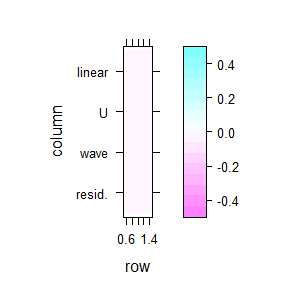levelplot(JP, what = "projections", main = "projections")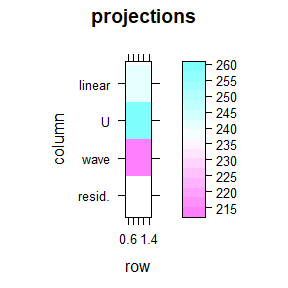These two functions take a JPtest object as argument and a character vector as what argument that allows to choose what is printed or plotted. It can take values “projections”, “statistics” or “pvalues” to display the associated dimension of the test entries in the inputted JPtest object.

## Working with several ensembles

If all the ensembles have the same number of members (such as in the ppensembles data set) one can process them in one go.

ppensembles <- ppensembles
fcsts <- lapply(ppensembles, function(x) x[, 4:33, with =FALSE])
obss <- lapply(ppensembles, function(x) t(x[, 34, with =FALSE]))
rkhists <- rkhist(fcsts, obss)
print(rkhists)
#>         rank
#> forecast  1  2  3  4  5  6  7  8  9 10 11 12 13 14 15 16 17 18 19 20 21 22 23
#>     CWAO 23 20 19 19 24 25 29 19 22 17 19 27 30 29 28 21 18 19 19 21 20 35 22
#>     DEMS 36 33 24 23 15 17 22 20 31 23 15 24 20 14 21 26 25 18 24 23 28 25 29
#>     ECMF 53 32 17 18 17 16 17 13 14  8 24 27 22 29 24 24 25 30 21 32 26 28 27
#>     EGRR 31 30 21 19 29 26 17 15 22 20 22 26 29 26 22 26 22 14 14 27 26 18 23
#>     RKSL 32 22 23 37 19 17 26 15 18 20 30 17 26 29 22 11 31 30 23 22 21 17 21
#>         rank
#> forecast 24 25 26 27 28 29 30 31
#>     CWAO 16 20 11 13 25 29 29 63
#>     DEMS 21 24 28 32 25 24 17 24
#>     ECMF 21 30 19 18 23 20 23 33
#>     EGRR 27 21 23 27 18 30 24 36
#>     RKSL 28 27 22 32 18 17 20 38
plot(rkhists, what = "percents")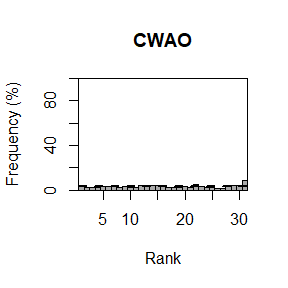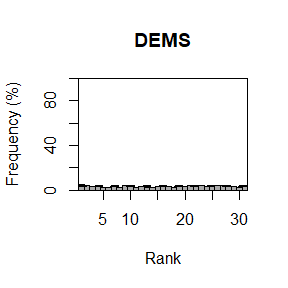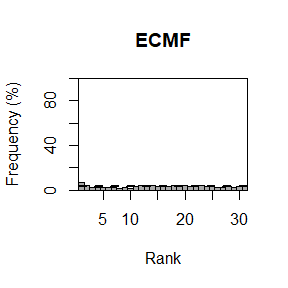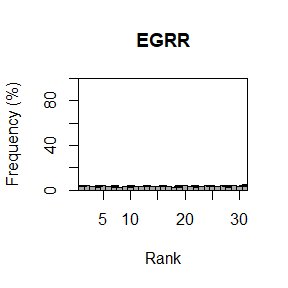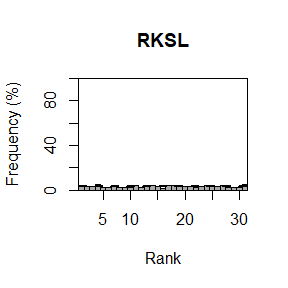plot(rkhists)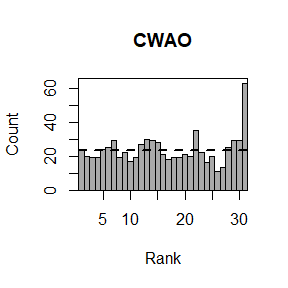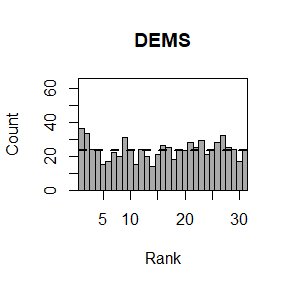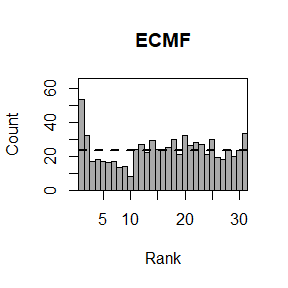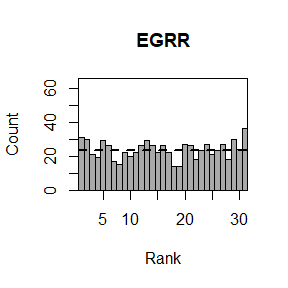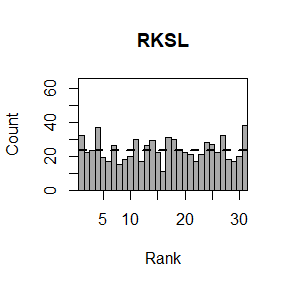deviates <- get_deviates(k = NCOL(rkhists))
ppJP <- JP_test(rkhists, deviates)
levelplot(ppJP, main = "pvalues")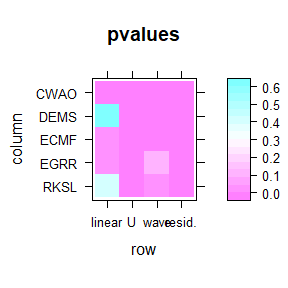levelplot(ppJP, what = "projections", main = "projections")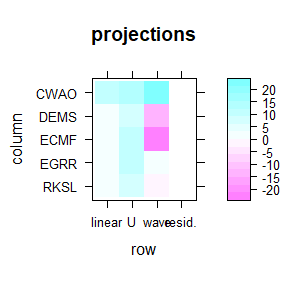# Flatness indices

Wilks (2019) compared several indices computed from rank histograms that have been proposed to assess whether a rank histogram is flat. These indices can be computed with the S3 generic function flatness_indices of the flatness package.

indices <- flatness_indices(rkhists)
print(indices)
#>          chisq        RI   entropy
#> CWAO 104.47332 0.2463263 0.9828671
#> DEMS  36.02736 0.1672477 0.9927921
#> ECMF  85.05062 0.2470323 0.9840062
#> EGRR  35.43365 0.1813689 0.9928062
#> RKSL  54.17784 0.2271744 0.9893028

Function flatness_indices takes as argument a matrix containing (rank) histograms in each row (by default, unless the argument col_wise is set to TRUE). It returns a matrix with three columns and as many rows as inputted histograms. The three columns correspond to the tree computed flatness indices the $$\chi^2$$ statistics, the reliability index and the entropy. Please refer to Wilks (2019) and references therein for further details on the last two indices.